# pytorch 的 C++ extension 写法

###### 本文授权转自知乎作者Monstarrrr，https://zhuanlan.zhihu.com/p/100459760。未经作者许可，不可二次转载。

2019年的最后一天了，终于填了一个早就想了解的坑。就是关于pytorch如何自定义一个扩展，这里主要是说C++扩展。

pytorch的C++ extension和python的c/c++ extension其实原理差不多，本质上都是为了扩展各自的功能，当然也为了使程序运行更加有效率，差别在于pytorch的C++ extension实施步骤较python的c/c++ extension的要简化一些。

• 利用C++写好自定义层发功能，主要包括前向传播和方向传播，以及pybind11的内容。
• 写好setup.py脚本， 并利用python提供的setuptools来编译并加载C++代码。
• 编译安装，在python中调用C++扩展接口

pybind11是python的一个库，主要负责python与C++11之间的通信

/*test.h*/
#include <torch/extension.h>
#include <vector>

// forward propagation
torch::Tensor Test_forward_cpu(const torch::Tensor& inputA, const torch::Tensor& inputB);
// backward propagation
std::vector<torch::Tensor> Test_backward_cpu(const torch::Tensor& gradOutput);

/*test.cpp*/
#include "test.h"

// part1:forward propagation
torch::Tensor Test_forward_cpu(const torch::Tensor& x, const torch::Tensor& y)
{
AT_ASSERTM(x.sizes() == y.sizes());
torch::Tensor z = torch::zeros(x.sizes());
z = 2 * x + y;
return z;
}

//part2:backward propagation
{
}

// part3:pybind11 （将python与C++11进行绑定， 注意这里的forward，backward名称就是后来在python中可以引用的方法名）
PYBIND11_MODULE(TORCH_EXTENSION_NAME, m){
m.def("forward", &Test_forward_cpu, "Test forward");
m.def("backward", &Test_backward_cpu, "Test backward");
}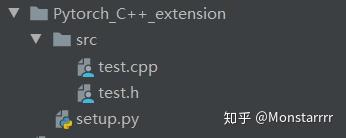setup.py中的内容为：

from setuptools import setup
import os
import glob
from torch.utils.cpp_extension import BuildExtension, CppExtension

# 头文件目录
include_dirs = os.path.dirname(os.path.abspath(__file__))
#源代码目录
source_file = glob.glob(os.path.join(working_dirs, 'src', '*.cpp'))

setup(
name='test_cpp',  # 模块名称
ext_modules=[CppExtension('test_cpp', sources=source_file, include_dirs=[include_dirs])],
cmdclass={
'build_ext': BuildExtension
}
)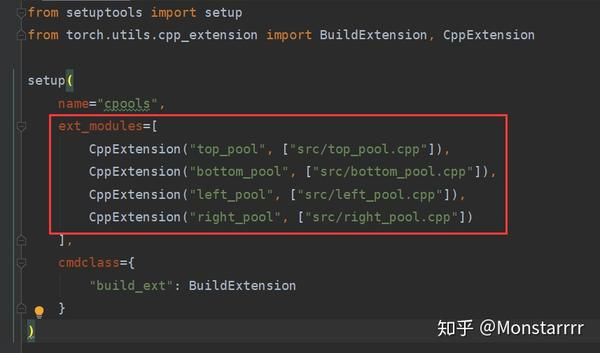NOTE:建议将扩展安装在个人虚拟环境中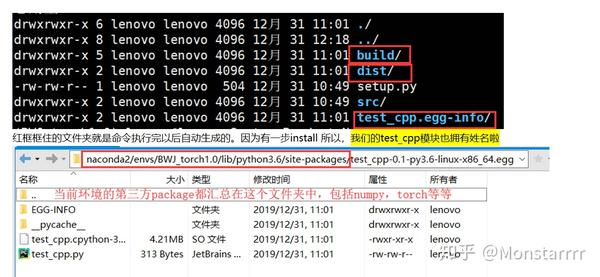NOTE：此时如果在python的控制台中输入import test_cpp会得到这样的错误：

from torch.autograd import Function
import torch
import test_cpp

class _TestFunction(Function):
@staticmethod
def forward(ctx, x, y):
"""
It must accept a context ctx as the first argument, followed by any
number of arguments (tensors or other types).
The context can be used to store tensors that can be then retrieved
during the backward pass."""
return test_cpp.forward(x, y)

@staticmethod

# 封装成一个模块（Module）
class Test(torch.nn.Module):
def __init__(self):
super(Test, self).__init__()

def forward(self, inputA, inputB):
return _TestFunction.apply(inputA, inputB)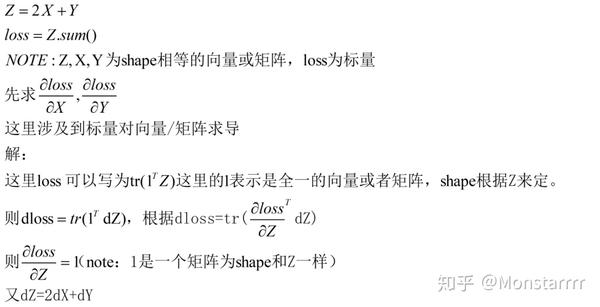page1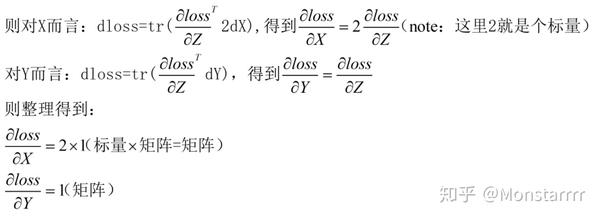page2

###########################END########################

All your lights are red， but I'm green to go.